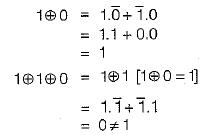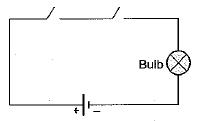Courses

# Boolean Algebra And Logic Gates (Basic Level - 1)

## 10 Questions MCQ Test Mock Test Series - Computer Science Engg. (CSE) GATE 2020 | Boolean Algebra And Logic Gates (Basic Level - 1)

Description
This mock test of Boolean Algebra And Logic Gates (Basic Level - 1) for Computer Science Engineering (CSE) helps you for every Computer Science Engineering (CSE) entrance exam. This contains 10 Multiple Choice Questions for Computer Science Engineering (CSE) Boolean Algebra And Logic Gates (Basic Level - 1) (mcq) to study with solutions a complete question bank. The solved questions answers in this Boolean Algebra And Logic Gates (Basic Level - 1) quiz give you a good mix of easy questions and tough questions. Computer Science Engineering (CSE) students definitely take this Boolean Algebra And Logic Gates (Basic Level - 1) exercise for a better result in the exam. You can find other Boolean Algebra And Logic Gates (Basic Level - 1) extra questions, long questions & short questions for Computer Science Engineering (CSE) on EduRev as well by searching above.
QUESTION: 1

Solution:
QUESTION: 2

Solution:
QUESTION: 3

### Which of the following logic expression is incorrect?

Solution:

The expression:Hence, (b) is the correct option.

QUESTION: 4

Let X and Y be the input and Z be the output of a AND gate. The value of Z is given by

Solution:
QUESTION: 5

What logic gate is represented by the circuit shown below:Solution:
QUESTION: 6

The boolean expression A + BC equals

Solution:

A + BC represents the distributive law which can be expanded as A + BC = (A + B) . (A + C)

QUESTION: 7

A positive AND gate is also a negative

Solution:
QUESTION: 8

A gate in which all inputs must be low to get a high output is called a/an

Solution:
QUESTION: 9

Logic 1 in +ve logic system is represented by

Solution:
QUESTION: 10

An AND circuit

Solution: